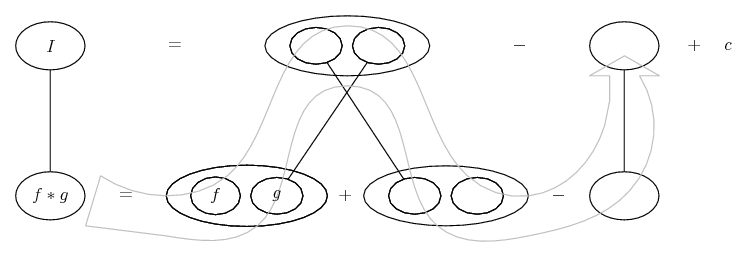1. ## integration4

integral(5(x^4)+4(x^5))/(((x^5+x+1))^2)

2. Is this your integral : $\displaystyle \displaystyle \int \frac{5x^4+5x^4}{(x^5+x+1)^2} \, dx$ ?

3.Originally Posted by GeneralIs this your integral : $\displaystyle \displaystyle \int \frac{5x^4+5x^4}{(x^5+x+1)^2} \, dx$ ?
the other is 4(x^5)

4. Bit of give and take in the numerator...

$\displaystyle \int \frac{5x^4 + 4x^5}{(x^5 + x + 1)^2}$

$\displaystyle =\ \int \frac{5x^4 + 4x^5 - (x^5 + x + 1) + (x^5 + x + 1)}{(x^5 + x + 1)^2}$

$\displaystyle =\ \int \frac{5x^4 + 5x^5 + x + 1 - (x^5 + x + 1)}{(x^5 + x + 1)^2}$

$\displaystyle =\ \int \frac{5x^4 (1 + x) + x + 1 - (x^5 + x + 1)}{(x^5 + x + 1)^2}$

$\displaystyle =\ \int \frac{(5x^4 + 1)(1 + x) - (x^5 + x + 1)}{(x^5 + x + 1)^2}$

Do parts on

$\displaystyle =\ \int \frac{(5x^4 + 1)}{(x^5 + x + 1)^2} (1 + x)$

(integrating the fraction)...

Edit: Just in case a picture helps...... where (key in spoiler) ...

Spoiler:... is the chain rule. Straight continuous lines differentiate downwards (integrate up) with respect to the main variable (in this case x), and the straight dashed line similarly but with respect to the dashed balloon expression (the inner function of the composite which is subject to the chain rule).

But this is wrapped inside the legs-uncrossed version of...... the product rule, where, again, straight continuous lines are differentiating downwards with respect to x.... is lazy integration by parts, doing without u and v.

Larger

_________________________________________

Don't integrate - balloontegrate!

Balloon Calculus; standard integrals, derivatives and methods

Balloon Calculus Drawing with LaTeX and Asymptote!

5. What a strange integral... considering the amount of trouble you have to go through to solve it (using any "normal" method) you'd think this can't have a nice solution but it has (I used Mathematica - sorry if that feels for you like I cheated) and wound up with something that was... well, funny how "nice" it was.

Anyway, all you have to do is note that the form tom@ballooncalculus wrote can be rewritten as:

$\displaystyle \int \frac{(5x^4+1)(x+1)-(x^5+x+1)}{(x^5+x+1)^2}\,dx$

$\displaystyle =-\int \frac{(x+1)'(x^5+x+1)-(x+1)(x^5+x+1)'}{(x^5+x+1)^2}\,dx$

And that is practically the definition of the quotient rule, so:

$\displaystyle =-\int(\frac{x+1}{x^5+x+1})'\,dx=-\frac{x+1}{x^5+x+1}$

Of course you get the same result by substituting

$\displaystyle t=\frac{x+1}{x^5+x+1}$.

Now, since any other way I can think of would require evaluating
$\displaystyle \int\frac{\,dx}{x^5+x+1}$, I guess this is the way it's supposed to be solved. I also congratulate anyone who would have been able to do that.

6. one might guess, because the numerator is a square, that the integral may be some quotient.

differentiating $\displaystyle \frac{f(x)}{x^5+x+1}$ gives:

$\displaystyle \frac{(x^5+x+1)f'(x) - f(x)(5x^4 + 1)}{(x^5+x+1)^2}$, which we want to be equal to $\displaystyle \frac{4x^5+5x^4}{(x^5+x+1)^2}$

that is, we want $\displaystyle (x^5+x+1)f'(x) - (5x^4 + 1)f(x) = 4x^5 + 5x^4$.

if f(x) is a polynomial, with leading term of degree n:

$\displaystyle f(x) = ax^n + r(x), f'(x) = nax^{n-1}+r'(x)$, then the leading term of

$\displaystyle (x^5+x+1)f'(x) - (5x^4 + 1)f(x)$ is $\displaystyle a(n-5)x^{n+4}$.

this tells us n = 1, and a = -1, that is f(x) = -x + b, for some real number b.

then $\displaystyle (x^5+x+1)f'(x) - (5x^4 + 1)f(x) = -x^5-x-1+5x^5+x-5bx^4-b$

$\displaystyle =4x^5-5bx^4-(b+1) = 4x^5+5x^4$, which only holds when b = -1.

we can thus conclude that f(x) = -x - 1

#### Search Tags

integration4